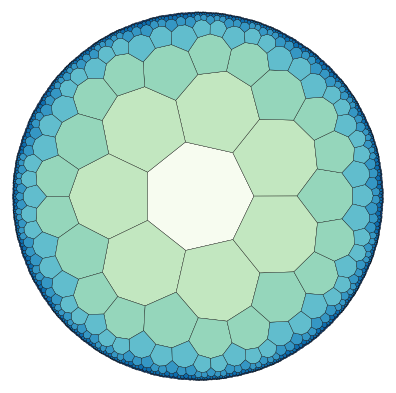# Quickstart

This Jupyter notebook demonstrates how a hyperbolic tiling can be constructed and plotted using only very few lines of code.

Import tiling object from hypertiling library

:

from hypertiling import HyperbolicTiling


Set parameters and generate the tiling

:

p = 7
q = 3
nlayers = 5

T = HyperbolicTiling(p,q,nlayers)


The size of the tiling can be queried like this

:

print("Number of cells:", len(T))

Number of cells: 232


Import and use available script for a quick plot

:

from hypertiling.graphics.plot import plot_tiling

import matplotlib.cm as cmap
import numpy as np

:

colors = np.random.rand(len(T))

plot_tiling(T, colors, cmap=cmap.RdBu, edgecolor="k", linewidth=0.2);By default, tilings are centered around a “cell”. They can also be centered around a vertex at the origin, using the following syntax

:

p = 4
q = 5
nlayers = 4

T = HyperbolicTiling(p, q, nlayers, center="vertex")


This example also demonstrates how keyword arguments like “clim” can easily be passed through the plot function

:

plot_tiling(T, np.random.rand(len(T)), cmap=cmap.OrRd, edgecolor="w", lw=1, clim=[-1,2]);In the next example we demonstrate how one can easily iterate over the cells of a tiling, e.g. in order to access their attributes

:

T = HyperbolicTiling(7, 3, 7, center="cell")


Paint cells by number of layer in which they reside

:

colors = []
for i in range(len(T)):
colors.append(T.get_layer(i))

plot_tiling(T, colors, cmap=cmap.GnBu, edgecolor="k", lw=0.2);Finally, something which just looks nice :)

:

T = HyperbolicTiling(3, 12, 4, center="vertex")

:

plot_tiling(T, np.random.rand(len(T)), cmap=cmap.OrRd, edgecolor="w", lw=0.4, clim=[-1,2]);The colors are a bit bright. Let’s make them more transparent.

:

plot_tiling(T, np.random.rand(len(T)), cmap=cmap.OrRd, edgecolor="w", lw=0.4, clim=[-1,2], alpha=0.5);